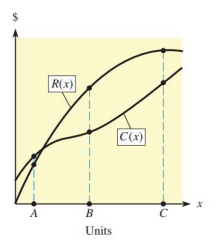# The graph shows the total revenue and total cost functions for a company. Use the graph to decide (and justify) at which of points A , B , and C profit is the greatest. there is a loss. producing and selling another item will increase profit. the next item sold will decrease profit.### Mathematical Applications for the ...

11th Edition
Ronald J. Harshbarger + 1 other
Publisher: Cengage Learning
ISBN: 9781305108042

#### Solutions

Chapter
Section### Mathematical Applications for the ...

11th Edition
Ronald J. Harshbarger + 1 other
Publisher: Cengage Learning
ISBN: 9781305108042
Chapter 9, Problem 15T
Textbook Problem
4 views

## The graph shows the total revenue and total cost functions for a company. Use the graph to decide (and justify) at which of points A, B, and C profit is the greatest. there is a loss. producing and selling another item will increase profit. the next item sold will decrease profit.This textbook solution is under construction.

### Still sussing out bartleby?

Check out a sample textbook solution.

See a sample solution

#### The Solution to Your Study Problems

Bartleby provides explanations to thousands of textbook problems written by our experts, many with advanced degrees!

Get Started